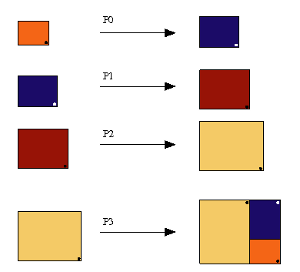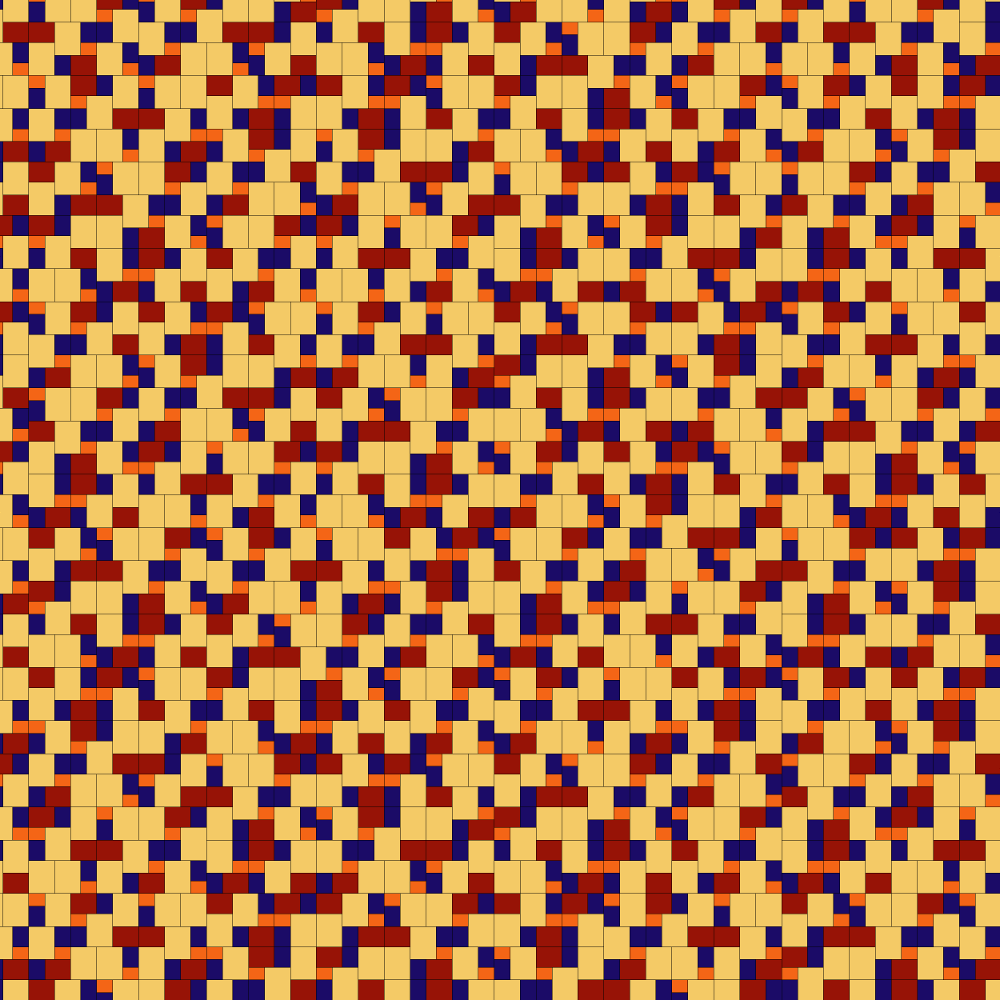### Info

Imagine a rectangle which can be decomposed into three proportional rectangles. The three rectangles have different sizes. The smallest one has the same orientation as the primitive rectangle. The other two rectangles have a 90 degrees with respect to the large rectangle. Starting with the smallest rectangle, the small side is 1 and the long side is x [where x is the square root of the golden number $\frac{\sqrt{5}+1}{2}$]. The next rectangle has x as a lower side. In order to keep the right proportion, its longest side should measure $x^2$. For the other rectangles proceed in same way.

### Substitution Rule### Patchdownload vectorformat Rectangulo dorado